# F-Mount

Lens mount developed by Nikon, typical used for line scan cameras

# F-Theta

F-Theta lenses are a class of Fisheye lenses

F-Theta lenses are also called “equidistant“, “linear scaled“, “äquidistant” or “angular
F-Theta lenses use an image mapping function of type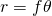Non distortion 180° F-Theta lenses have an image circle ormm
A non distortion F-Theta lens of 1.37mm focal length has an image circle of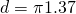mm =mm
Therefor a f=3.17mm F-Theata lens with an image diameter for 180° of 4.15mm has a distortion of (4.15/4.3)-1 = 0.965-1 = -3.5%

maintains angular distances

[table caption=”F-Theta angles” width=”500″ colwidth=”50|50|50|50″ colalign=”center|center|center|center”]
weak,medium,strong,max
115,159,217,360 and more
[/table]# far point

Most distant point on the optical axis with an image of “acceptable sharpness”Where CoC is the Circle of Confusion (the largest accepted Airy-disk) in Millimeter.

Alternatively, we can express the FarPoint using the magnification M :If we use for example a 1/2.5″ 5 Aptina Megapixel greyscale Sensor mit 2.2pixel pitch, we can use the pixel diagonal as CoC for crisp images, sayA 5 Mega lens with f=7.2mm focal length and F-stop F2.4, focused to an object distance of 100mm then has a far point ofund einen Nahpunkt von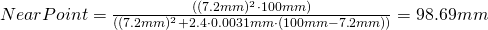and thusIf instead we use a 5 Megapixel greyscale Sony Sensor with 3.45pixel pitch, we can choose as CoC the diagonal of the pixel for crisp images, sayA 5 Mega lens with f=7.2mm focal length and F-stop F2.4, focussed to 100mm then results inmm
und einen Nahpunkt vonthus we getIf we use a color sensor instead we can usefor crisp images. For the two sensors above we then get: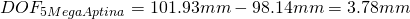To increase the DOF we can increase the Pixel Size, but we either lose resolution, or (at the same pixel count) the magnification changes)
If you change the focal length of a lens in a way, that (with the same sensor) you get the same FOV (then from a different distance) this results in the same DOF !!!
When a lens is focussed to the hyperfocal distance H, the far point is atand the near point is at.
The DOF isthen, thus focussing to the hyperfocal distance results in the largest possible DOF.

# Fermat’s Principle

If light travels from one point to another, it takes paths of a length that in first approximation is equal to the length of optical paths length closely adjascent to the actual path.

from one point to another light always chooses a path which’s OPL does not change if the path slightly varies.
Light always takes the shortest path in terms of speed but in general not the path of shortest distance.

The actual path taken has either a maximum or a minimum OPL compared to adjascent optical paths or is equal to the OPL of adjascent paths.

# Field of View

(usually horizontal) extend of an object that’s visible on a sensor.

Sometimes the HFOV (horizontal field of view), sometimes the VFOV (Vertical Field of View) and sometimes the DFOV (Diagonal Field of View) matters. It’s of utmost importance to state clearly which matters.

# Fisheye Types

Various types of fisheye lenses are available.
Here a short overview:

## Fisheye Types:

Type gnomonic stereographic F-Theta equal area orthographic
lens class wide angle Fisheye Fisheye Fisheye Fisheye
mapping function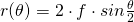normalized mapping function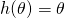meridional scaling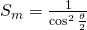sagittal scaling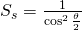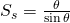effective scalingN from2 1 0 -1Balance Deform. vs. Scaling0 -22
Curvature0-1
Deformationmaintains angles angular distances areas planar illuminance
AOV<180° <360° >= 360° 360° 180°54° 94° 115° 94° 66°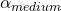75° 131° 159° 131° 90°102° 180° 217° 180° 120°

Source of the various angles and formulae: wikipedia

Here the angle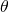is measured in Radians !

equal area lenses are also called “equisolid angle” or “flächentreu”
F-Theta lenses are also called “equidistant“, “linear scaled“, “äquidistant” or “angular
sterographic lenses are also called “ conform“or “ winkeltreu
orthographic lenses are also called” hemispherical“or “orthographisch

For details see:
equal area
F-Theta
gnomonic
orthographic
stereographic

# focal length

The focal length is the distance from the Image side principal plane to the image of objects at infinity.

For single lenses in air that is equal to the distance from the first focal point to the first principal point.
(in each case measured from the left to the right)

Note that this is a positive value for converging lenses and a negative value for the divergent lenses.

The larger the focal length, the smaller the aperture angle of the lens and the smaller the object section that is displayed full-frame on the sensor.
The lens captures less of the object. Extremea are telephoto lenses and finally telescopes.

The smaller the focal length, the larger the aperture angle of the lens and the larger the object section which is displayed full-frame on the sensor.
The lens captures more of the object. Extreme forms are fisheye lenses.

Lenses are typically listed, sorted by focal length. As an approximation, lenses with larger focal length see a smaller portion of the object (in more detail).

There are exceptions! (See: pseudo-knowledge: viewing angle and focal length are equivalent)

The following calculators determine focal lengths. They assume, a pin hole lens model, the Chief Ray Angles are assumed to be the same on object and image side.
Therefore for wide angles a too small focal length is returned .. (as all focal length calculators on the internet do 😉 );

Also keep in mind that the Gauss lens equation and the Newtonian image equation both assume same CRA on object and image side, which is nearly never given for wide angle lenses and for sure not for fisheye lenses.

Therefore when you calculate focal length, object and image distances, they will in general differ from the real world situation you measure!

The following calculator determines focal length from angles.
However, Viewing angles change with the working distance! Also, a Pinhole lens model is assumed. Thus for wide angles a too small focal length is returned .. (as all focal length calculators on the internet do 😉 )

For the next calculator it is very important to correct the distortions before doing the calculation: